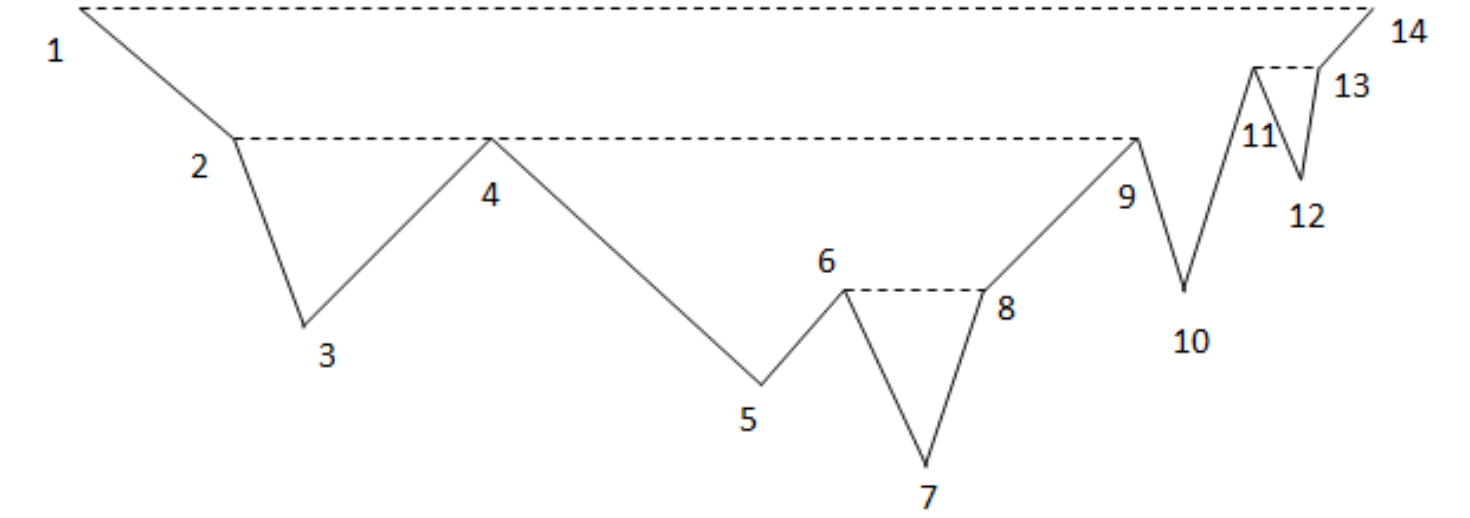﻿ Ningbo University of Technology Online Judge ::  Aerial Tramway
• ###  Aerial Tramway

• 时间限制: 5000 ms　内存限制: 65535 K
• 问题描述
• An aerial tramway, cable car, ropeway or aerial tram is a type of aerial lift which uses one or two stationary ropes for support while a third moving rope provides propulsion. With this form of lift, the grip of an aerial tramway cabin is fixed onto the propulsion rope and cannot be decoupled from it during operations.

-- WikipediaYou own a park located on a mountain, which can be described as a sequence of n points (xi, yi) from left to right, where xi,yi>0, xii+1, yi!=yi+1 (that means there will not be horizontal segments in the mountain skyline), illustrated below(the numbers are the corresponding x-coordinate):Since the mountain is very sloppy, some aerial tramways across the park would be very helpful. In the figure above, people can go from p4 to p9 directly, by taking a tram. Otherwise he must follow a rather zigzag path: p4-p5-p6-p7-p8-p9.

Your job is to design an aerial tramway system. There should be exactly m trams, each following a horizontal segment in the air, between two points pi and pj. "Horizontal" means yi=yj, in the air" means all the points in between are strictly below, i.e. yki for every i2 and p9, because p4 is not strictly below p2-p9. However, you can have two trams, one from p2 to p4, and one p4 to p9. There is another important restriction: no point can be strictly below k or more tramways, because itll be dangerous. For example, if k=3, we cannot build these 3 tramways simultaneously: p1-p14, p4-p9, p6-p8, because p7 would be dangerous.

You want to make this system as useful as possible, so you would like to maximize the total length of all tramways. For example, if m=3, k=3, the best design for the figure above is p1-p14, p2-p4 and p4-p9, the total length is 20. If m=3, k=2, you have to replace p1-p14 with p11-p13, the total length becomes 9.

• 输入
• There will be at most 200 test cases. Each case begins with three integers n, m and k(1<=n,m<=200, 2<=k<=10), the number of points, the number of trams in your design and the dangerous parameter introduced earlier. The next line contains n pairs of positive integers xi and yi.(1<=xi,yi<=105).
• 输出
• For each test case, print the case number and the maximal sum. If it is impossible to have exactly m tramways, print -1.
• 样例输入
• ```14 3 3
1 8
2 6
3 4
4 6
5 3
6 4
7 1
8 4
9 6
10 4
11 6
12 5
13 6
14 8
14 3 2
1 8
2 6
3 4
4 6
5 3
6 4
7 1
8 4
9 6
10 4
11 6
12 5
13 6
14 8```
• 样例输出
• ```Case 1: 20
Case 2: 9```
• 提示
• `无`
• 来源
• `第十一届“蓝狐网络杯”湖南省大学生计算机程序设计竞赛`
• 操作
﻿